• +91 9971497814
• info@interviewmaterial.com

# Chapter 12 Introduction to Three Dimensional Geometry Ex-12.3 Interview Questions Answers

### Related Subjects

Question 1 : Find the coordinates of the point which divides the line segment joining the points (–2, 3, 5) and (1, –4, 6) in the ratio (i) 2:3 internally, (ii) 2:3 externally.

(i) The coordinates ofpoint R that divides the line segment joining points P (x1y1z1) and Q (x2y2z2) internally in the ratio mare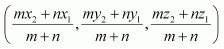.

Let R (x, yz) be the point that divides the linesegment joining points(–2, 3, 5) and (1, –4, 6) internally in the ratio 2:3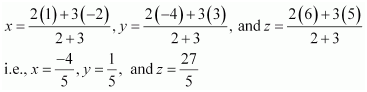Thus, thecoordinates of the required point are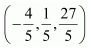.

(ii) Thecoordinates of point R that divides the line segment joining points P (x1y1z1) and Q (x2y2z2) externally in the ratio mare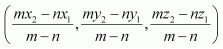.

Let R (x, yz) be the point that divides the linesegment joining points(–2, 3, 5) and (1, –4, 6) externally in the ratio 2:3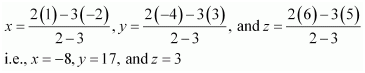Thus, thecoordinates of the required point are (–8, 17, 3).

Question 2 : Given that P (3, 2, –4), Q (5, 4, –6) and R (9, 8, –10) are collinear. Find the ratio in which Q divides PR.

Let point Q (5, 4, –6)divide the line segment joining points P (3, 2, –4) and R (9, 8, –10) in theratio k:1.

Therefore,by section formula,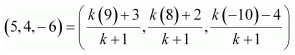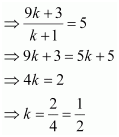Thus,point Q divides PR in the ratio 1:2.

Question 3 : Find the ratio in which the YZ-plane divides the line segment formed by joining the points (–2, 4, 7) and (3, –5, 8).

Let the YZ plane divide theline segment joining points (–2, 4, 7) and (3, –5, 8) in the ratio k:1.

Hence, bysection formula, the coordinates of point of intersection are given by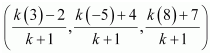On the YZplane, the x-coordinate of any pointis zero.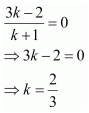Thus, theYZ plane divides the line segment formed by joining the given points in theratio 2:3.

Question 4 : Using section formula, show that the points A (2, –3, 4), B (–1, 2, 1) and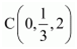are collinear.

The given points are A (2,–3, 4), B (–1, 2, 1), and.

Let P bea point that divides AB in the ratio k:1.

Hence, bysection formula, the coordinates of P are given by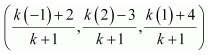Now, wefind the value of k at whichpoint P coincides with point C.

By taking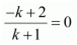, we obtain k = 2.

For k = 2, the coordinates of point P are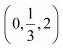.

i.e.,is a point that divides ABexternally in the ratio 2:1 and is the same as point P.

Hence,points A, B, and C are collinear.

Question 5 : Find the coordinates of the points which trisect the line segment joining the points P (4, 2, –6) and Q (10, –16, 6).

Let A and B be the pointsthat trisect the line segment joining points P (4, 2, –6) and Q (10, –16, 6)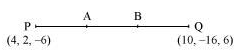Point Adivides PQ in the ratio 1:2. Therefore, by section formula, the coordinates ofpoint A are given by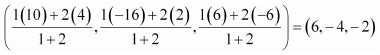Point Bdivides PQ in the ratio 2:1. Therefore, by section formula, the coordinates ofpoint B are given by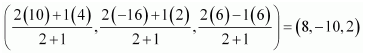Thus, (6,–4, –2) and (8, –10, 2) are the points that trisect the line segment joiningpoints P (4, 2, –6) and Q (10, –16, 6).

Todays Deals### Chapter 12 Introduction to Three Dimensional Geometry Ex-12.3 Contributorskrishan

Name:
Email:

# Latest News# 9000 interview questions in different categories